Schwarz function

An analytic function realizing a conformal mapping of a triangle bounded by arcs of circles onto the upper half-plane (or unit disc) that remains single-valued under unrestricted analytic continuation. A Schwarz function is an automorphic function. The corresponding group depends on the form of the mapped triangle. The requirement of single-valuedness is satisfied only in the case when the angles of the triangle are,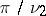,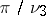, where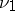,and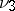are some specially-chosen natural numbers.
If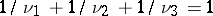, one obtains rectilinear triangles for which the only possibilities are: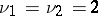,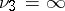(a semi-strip);,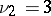,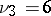;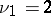,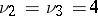;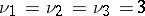. In all these cases the Schwarz functions are represented by trigonometric functions or Weierstrass elliptic functions and are automorphic; their group is the group of motions of the Euclidean plane.
If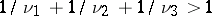, there are the following possibilities:,arbitrary;,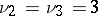;,,;,,. In all these cases the Schwarz functions are rational automorphic functions; their group is a finite group of motions of a sphere. As a result of the relationship between this group and regular polygons, such Schwarz functions are also called polyhedral functions.
Finally, if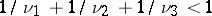, then infinitely-many different triangles are possible, sincemay increase indefinitely. Here, the Schwarz functions are automorphic functions with a continuous singular curve (circle or straight line). In particular, the cases of,,and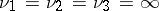(a circular triangle with zero angles) lead to the modular functions (cf. Modular function)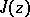and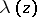, respectively. The Schwarz functions were studied by H.A. Schwarz .